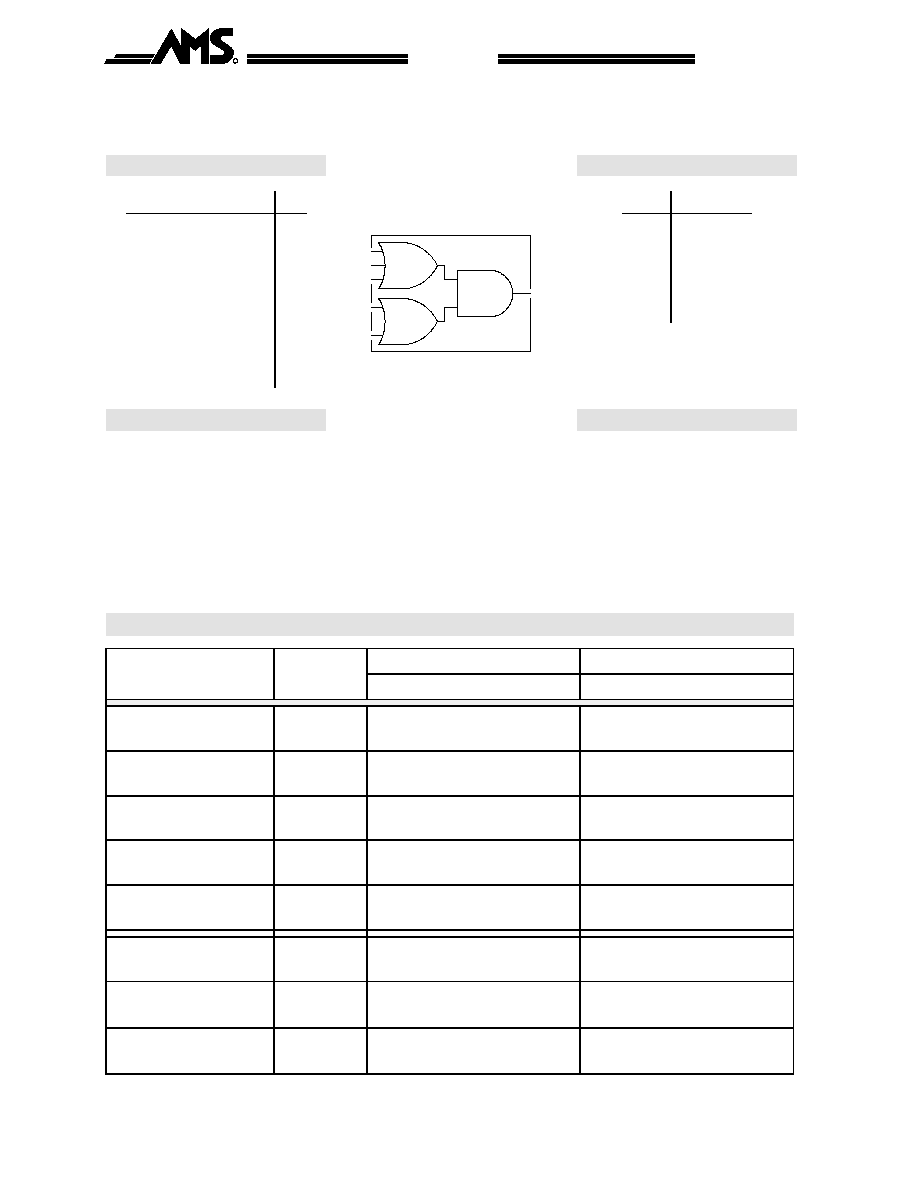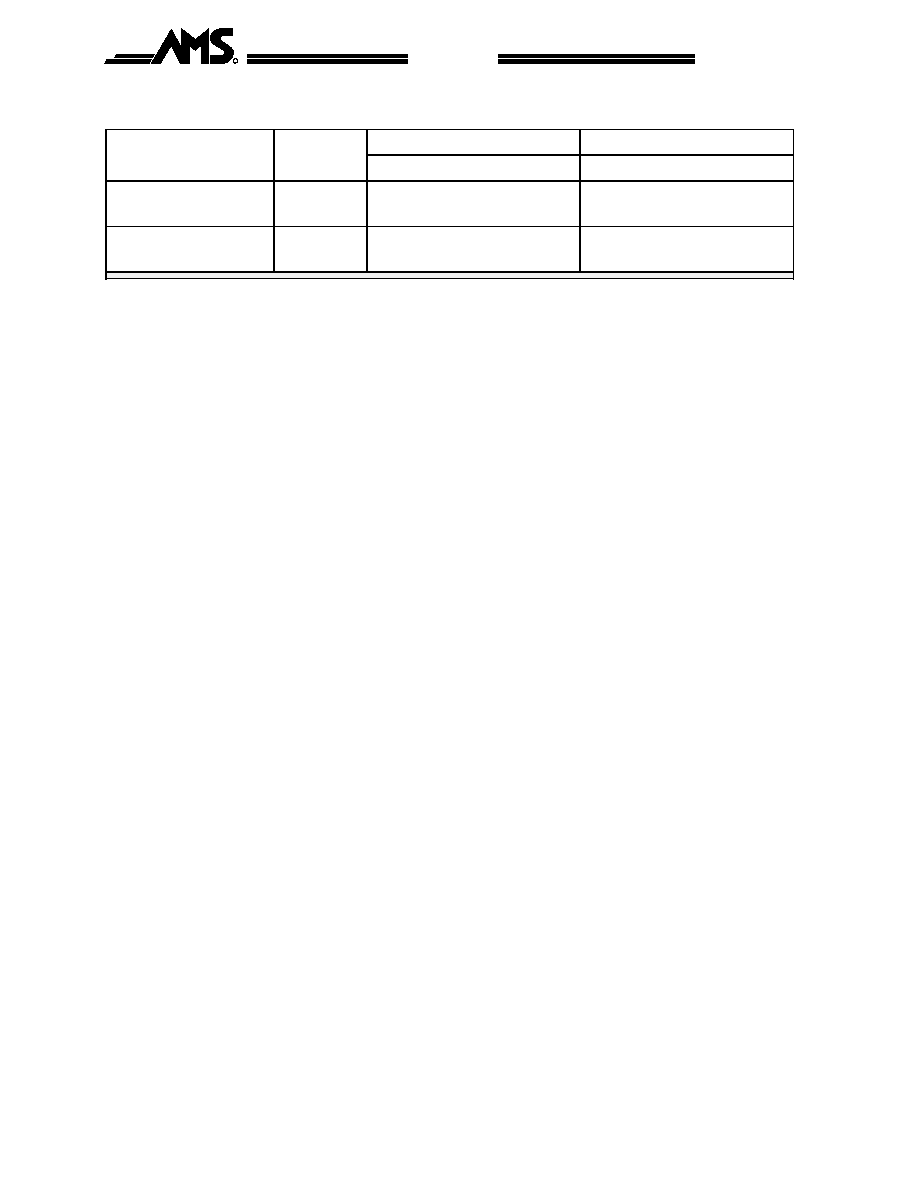–ü–æ–∏—Å–∫ –¥–∞—Ç–∞—à–∏—Ç–∞ (1.687.043 –∫–æ–º–ø–æ–Ω–µ–Ω—Ç–æ–≤) –ì–¥–µ –∏—Å–∫–∞—Ç—å–≤ –Ω–∞–∏–º–µ–Ω–æ–≤–∞–Ω–∏–∏–≤ –æ–ø–∏—Å–∞–Ω–∏–∏### Datasheet: oa32 (No company)

Is An Or / and Circuit Providing The Logical Function Q = [ (a+b+c).(d+e) ].
–°–∫–∞—á–∞—Ç—å:PDFZIPOA32 is an OR
/
AND circuit providing the logical function Q = [ (A+B+C).(D+E) ].
Truth Table
OA32
A
C
D
Q
E
B
Capacitance
A
B
C
D
E
Q
Ci (pF)
L
L
L
X
X
L
A
0.054
X
X
X
L
L
L
B
0.054
X
X
H
X
H
H
C
0.066
X
X
H
H
X
H
D
0.048
X
H
X
X
H
H
E
0.056
X
H
X
H
X
H
H
X
X
X
H
H
H
X
X
H
X
H
Area
Power
0.95 mils
2
2.72 µW
/
MHz

Delay [ns] = tpd.. = f(SL, L)
with SL = Input Slope [ns] ; L = Output Load [pF]
Output Slope [ns] = op_sl.. = f(L)
with L = Output Load [pF]
AC Characteristics : Tj = 25∞C VDD = 3.3V Typical Process
AC Characteristics
Characteristics
Symbol
SL = 0.1
SL = 2.0
L = 0.1
L = 0.7
L = 1.0
L = 0.1
L = 0.7
L = 1.0
Delay A to Q
tpdar
0.74
2.12
2.82
0.85
2.22
2.87
tpdaf
0.82
1.98
2.50
0.94
2.10
2.62
Delay B to Q
tpdbr
0.69
2.05
2.79
0.79
2.13
2.81
tpdbf
0.80
1.96
2.49
0.97
2.13
2.68
Delay C to Q
tpdcr
0.62
2.01
2.64
0.71
2.03
2.73
tpdcf
0.73
1.87
2.41
1.00
2.15
2.71
Delay D to Q
tpddr
0.69
2.09
2.75
0.88
2.27
2.91
tpddf
0.69
1.87
2.42
0.86
2.04
2.60
Delay E to Q
tpder
0.63
2.00
2.76
0.83
2.19
2.86
tpdef
0.66
1.82
2.36
0.94
2.12
2.67
Output Slope A to Q
op_slar
1.02
5.30
7.48
0.95
5.27
7.48
op_slaf
0.78
3.76
5.02
0.78
3.63
5.00
Output Slope B to Q
op_slbr
0.96
5.31
7.37
0.92
5.26
7.50
op_slbf
0.80
3.68
4.98
0.78
3.65
5.07
Output Slope C to Q
op_slcr
0.95
5.20
7.52
0.88
5.23
7.48
op_slcf
0.78
3.80
5.03
0.78
3.62
5.03
Sept. 1996
- 285 -
Rev. N/C
Austria Mikro S ysteme International
R
0.6 µm CMOS
CUB
OA32Characteristics
Symbol
SL = 0.1
SL = 2.0
L = 0.1
L = 0.7
L = 1.0
L = 0.1
L = 0.7
L = 1.0
Output Slope D to Q
op_sldr
1.01
5.33
7.55
0.93
5.23
7.50
op_sldf
0.80
3.77
5.10
0.81
3.78
5.08
Output Slope E to Q
op_sler
0.95
5.32
7.56
0.92
5.26
7.48
op_slef
0.77
3.62
5.00
0.83
3.78
5.17
Sept. 1996
- 286 -
Rev. N/C
Austria Mikro S ysteme International
R
0.6 µm CMOS
CUB
OA32